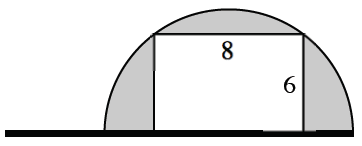### Home > APCALC > Chapter 3 > Lesson 3.4.3 > Problem3-181

3-181.

Calculate the volume of the solid created when the semi-circular flag at right is rotated about the pole. Describe the rotated solid.

This flag will look like a sphere with a cylindrical hole removed from its center.

$\text{volume} = \text{sphere} − \text{cylinder}$

$=\frac{4}{3}\pi r^{3}-\pi r^{2}h$

Use the Pythagorean Theorem to compute the radius of the sphere.